Smartick is an online platform for children to master math in only 15 minutes a day

May07

# Coordinates: What They Are Used for and Some Examples with Smartick

Cartesian coordinates are numbers that indicate the position of a point in a given space. In this case a two-dimensional space, a plane. René Descartes was the first mathematician to formally make use of them, which is where the name “Cartesian” comes from.

### How do Cartesian coordinates work?

If we want to indicate something on a plane, we need:

• A horizontal measurement: left-right. We call this X.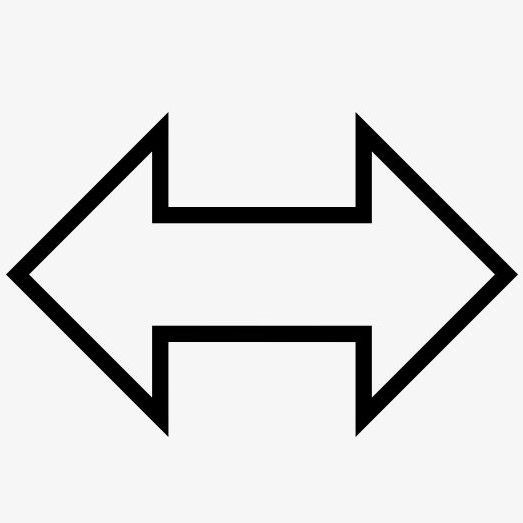• A vertical measurement: up-down. We call this Y.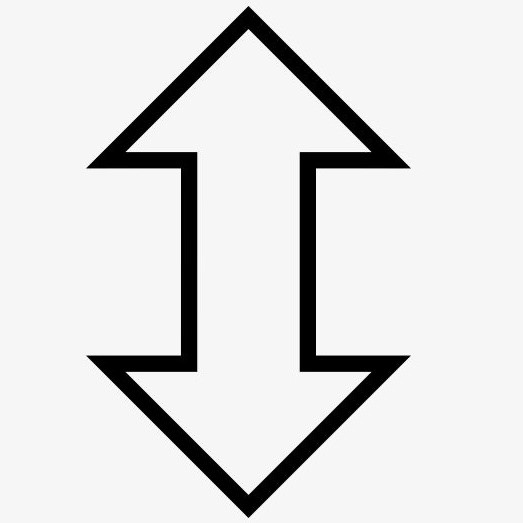• A reference point from which we can measure: the origen. We call this O.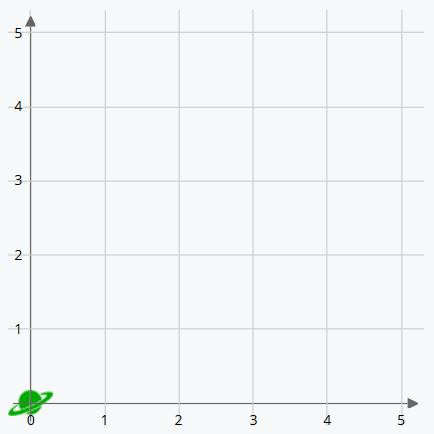O, the origen, is where you can see the green planet in the image above. It’s called the origen because it’s the point from which the lines that delineate the two axes of the coordinates originate.

Let’s look at the horizontal line, left-right. As you can see, the numbers start at the origen. The first value is zero and the numbers increase as we move to the right. This is the X axis of the coordinates, and the larger its value, the farther to the right the dot is placed. So, 4 on the X axis is 4 positions to the right of the origen.

We also have a vertical line, up-down. In the same way as the X axis, the values start at the origen with zero and increase as we move upwards. This is the Y axis of the coordinates. The larger its value, the higher we go. For example, 5 is five positions above the origen.

In the game “pin the tail on the donkey” we need the other players to tell us how far to the left or the right and how far up or down we need to move to pin the tail correctly. To indicate a point on a plane we need to do the exact same thing. We need to plot two numbers: one on the X axis and the other on the Y axis. This defines the position: the first number indicates how far to the right it is and the second number how far up it is. These numbers are usually separated by a comma and placed inside parentheses, like this: (X ,Y).

### Examples of coordinates

The origen is always situated at the coordinates (0,0). In other words, it’s the lowest point possible, and farthest to the left. O is a spatial point from which the axes of the coordinates originate and is “0 positions to the right and 0 positions up”. This is the point from which we start to count. Therefore, (0,3) will be 0 positions to the right and 3 up. And (5,0) will be 5 positions to the right and 0 up.

For example, a blue airplane is at the coordinates (3,2) Where will we find it?

The first coordinate we’re given is the position on the X axis. We have to count 3 positions to the right from the origen. The second coordinate is gives us the position on the Y axis, so we count 2 positions up. Therefore, we place the blue plane 3 positions to the right of the origen and 2 places above.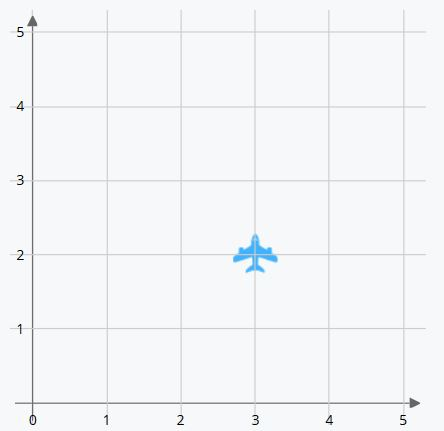Now let’s look at an inverse example. We place the object first and then indicate the coordinates of the position its in: What coordinates is the orange tree at?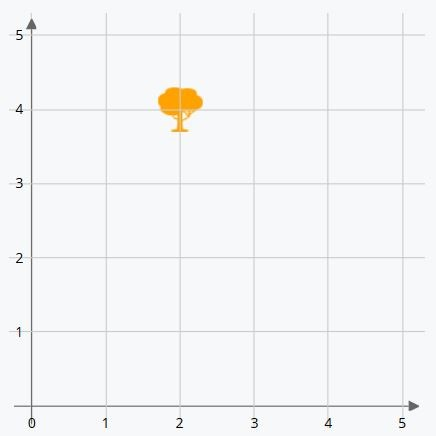We can see that it’s situated two positions to the right of the origen (X axis) and 4 above (Y axis). First we write the X axis coordinate and then the Y axis coordinate.

The orange tree is at the coordinates (2,4).

### What are coordinates used for?

Aside from its use in mathematics, we can find coordinates used on a daily basis to indicate places on maps. The map can show a few streets, a city, or the entire world. So you can find your friend’s house with a map of their city or even know exactly where you are with GPS if you’re in the middle of a wild jungle or a scorching desert.

Maps can be divided into sectors with X and Y axes. And people use GPS every day to get directions while driving or walking, or to work out how long it takes to get from one point to another. It’s a system that uses coordinates to find our position and the position of our destination.

### Coordinates in Smartick

Smartick works on activities like the ones in the examples we saw above.

The sequences of activities in Smartick are designed to help children first understand the basics of coordinates before increasing in complexity.

You can find these types of exercises in previous blog posts, for example here.